## Creating a Pulse Train in FullWAVE Using Custom Time Envelopes

Mode-locked lasers, which generate a train of ultra-short pulses, have become an important tool in precision optical frequency measurement  and are useful for the design of future ultrahigh-speed optical communication systems . This note will discuss how to use FullWAVE™'s custom time envelope feature to create this type of pulse train. Details about generating a custom time envelope can be found in Section 4.B.2 of the FullWAVE manual.  Users can access design files on the Customer Support Portal.

The output of a mode-locked laser is a comb of equidistant lines in the frequency domain, separated by a bandwidth of frep. In the time domain, this corresponds to a train of pulses whose temporal separation is given by the cavity round-trip time trt = 1/frep. The offset of the comb, fceo is connected to the pulse-to-pulse phase slip
Δϕ = 2π(fceo/frep). We are going to create a pulse train with fceo = frep/4 , such that every fifth pulse is identical, as shown in Figure 1 .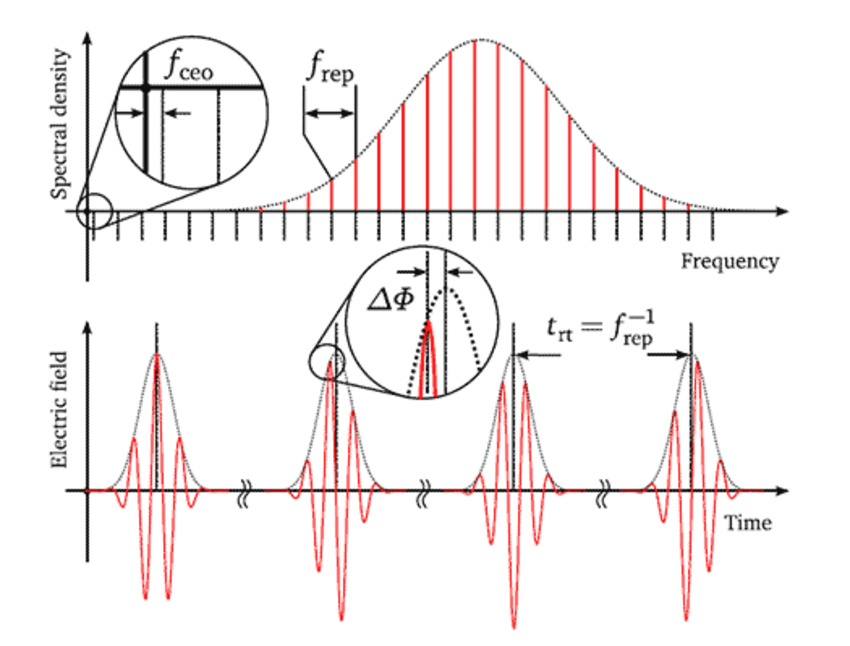Figure 1. Pulse Train with fceo = frep/4, such that every fifth pulse is identical

The electric field of such a pulse train can be written as: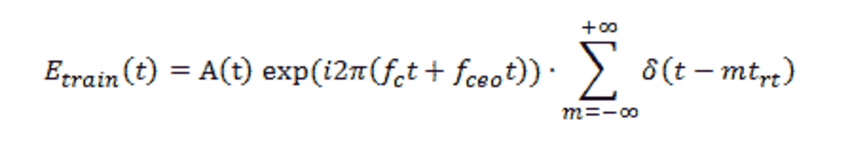(1)

where A(t) denotes the pulse envelope and fc c/λ is the central frequency of the comb and the function δ(t – mrrt) function produces the pulse train. Note that the time envelope equation for FullWAVE’s Pulse excitation type, as described in Section 4.D.2 of the FullWAVE manual, is: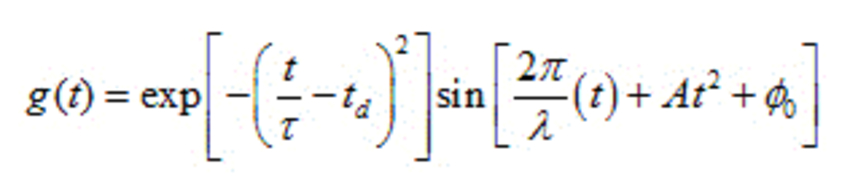(2)

The custom envelope in Eq.(1) can be written as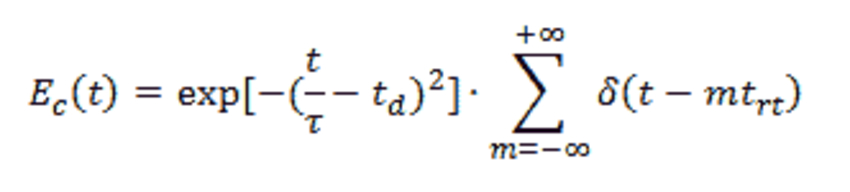(3)

And the excitation wavelength will be modified by fceo term and become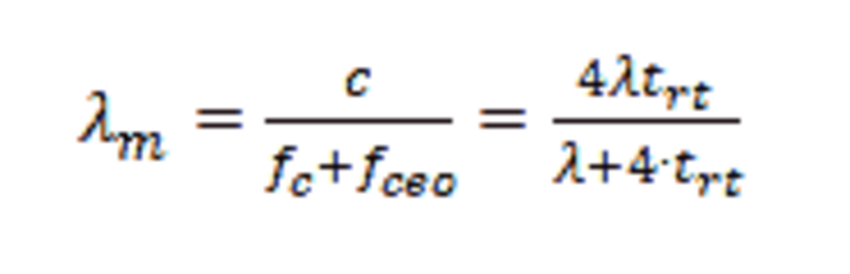(4)

Using the above analysis, a simple model has been created in the design file pulsetrain.ind. We created the following symbols to help with the definition:

 Symbol Value Description DC 0.6 Duty Cycle, set as ratio of one time period Lambda_c 0.5 Initial Center Wavelength Lambda_m 4*Lambda_c*Trt/(4*Trt+Lambda_c) Modified Launch Wavelength Trt 3 Pulse Repeating Time Tp Lambda_c/4 Pulse Time Td Lambda_c*6 Pulse Delay Time free_space_wavelength Lambda_c The global wavelength setting fdtd_excitation_envelope TIME_ENVELOPE_USER Enables the use of a custom time envelope fdtd_user_envelope exp(-((t/Trt-floor(t/Trt))/Tp-Td)^2)*step2((t/Trt-floor(t/Trt)),DC) Custom time envelope; the step2() function represents the repeating delta function and the floor() function is used to create the same envelope at each repeated time interval t 1 The variable t must be in the symbol table so that we can use it in the envelope function; The value does not matter

Note that we have also enabled the Excitation Time Waveform option in the Output Options dialog so that we can view the time envelope used in the simulation. The animated results of the FullWAVE simulation are shown in Figure 2 and the excitation envelope is shown in Figure 3. Red lines indicate the pulse center and the green curve shows the envelope of each pulse.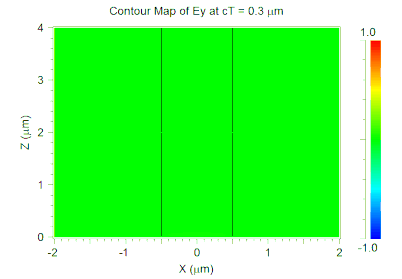Figure 2. Pulse train propagating in a waveguide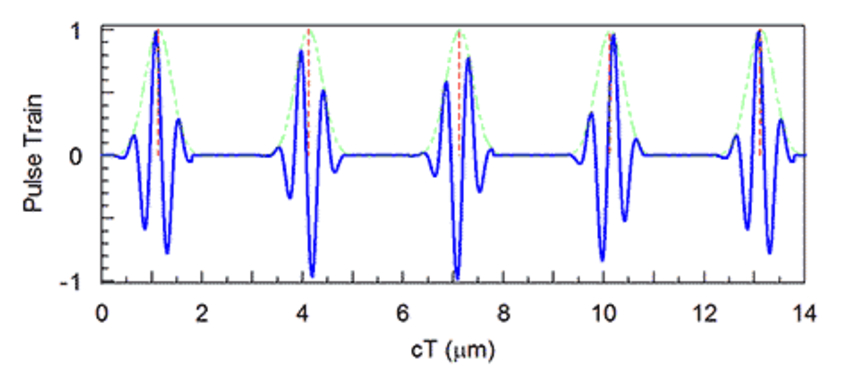Figure 3. The pulse train recorded as time waveform (.pstm file).
The red lines have been added as WinPLOT drawing options in this plot.